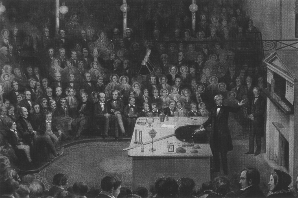# Natural Science - Year I

#### Unit 9: Determining velocity and accelerationLab Assignment for Unit 9

 This Unit's Homework Page History Lecture Science Lecture Lab Parents' Notes

## Goal: To measure velocity and acceleration in a simple system.

### Materials and Equipment:

• Ruler (preferably with a central grove) or other long, flat, ramp at least 12 inches (30 cm) long.
• Marble or other small smooth ball
• Clock with second hand

### Procedure:

1. Set up your ramp on a level surface. Raise one end of the ramp about 1.5 cm (no more than 1 inch).
2. Place the marble at the top of the ramp and release it with zero starting velocity.
3. Measure how far down the ramp the marble rolls in two seconds.
4. Repeat your experiment at least 4 times (5 total trials).
5. Repeat the entire experiment (another 5 trials), measuring how far the marble rolls in three seconds.

### Data Analysis:

1. Organize your data in a table like this one:
 Trial # Time (seconds) Distance (centimeters or inches) 1 2
2. Calculate the average distance the marble rolled in two seconds.
3. Calculate the average distance the marble rolled in three seconds.
4. Calculate the average change in speed (acceleration), if any.